## Quadratic Newton Iteration

Let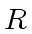be a commutative ring with units. Let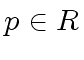and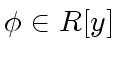. We are concerned with solving the equation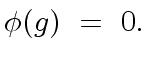(29)

The following Proposition 8 states that Relation (31) computes better and better approximations of solutions of Relation (29) that is, approximations modulo higher and higher powers of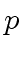. Comparing to the results of the previous section, this proposition offers a significant improvement: the accuracy doubles at each step, leading to the so-called Quadratic Symbolic Newton Iteration.

Proposition 8   Letand let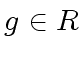be such that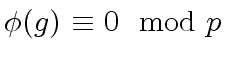(30)

Assume that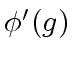is invertible moduloand let denote by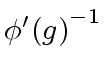its inverse. Consider an element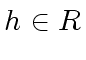such that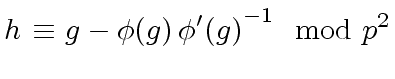(31)

Then the following assertions hold:
1.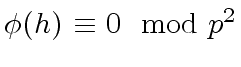,
2.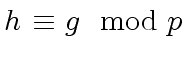,
3.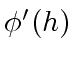is invertible modulo, and thus modulo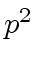.

Proof. Sinceis invertible modulothenis invertible modulo. (This is easy to check: Exercise!) Hence Relation 31 makes sense. In fact, Algorithmcomputes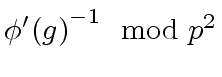given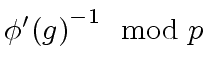.

Sincedivides, the following congruence holds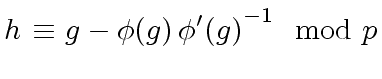(32)

Since, this leads to(33)

and we have proved the second claim. Let us prove the first one. Using the Taylor expansion of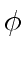at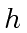and the fact thatdivides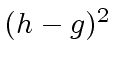we obtain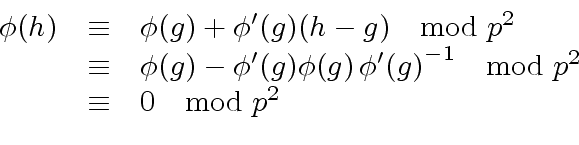(34)

proving the first claim. Finally, observe thatimplies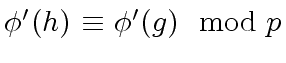. Indeed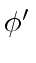is a polynomial in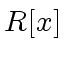. Therefore,is invertible modulo, sinceis invertible modulo, by hypothesis.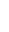Remark 7   Intuitively, Proposition 8 says that if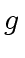is a good approximation of a zero ofthenis a better approximation, at least twice as accurate.

Remark 8   Proposition 8 leads to an algorithm provided that we can
• check whetheris invertible moduloand
• compute its inverse moduloin case.
Then for computing the inverse ofmodulothe idea is to apply the algorithm to itself! That is, to the particular case of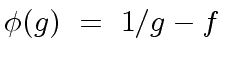(35)

This leads to Algorithm 1. Finally recall that for a prime elementin an Euclidean domain, the computation ofmodulocan be done by the Extended Euclidean Algorithm.

Algorithm 1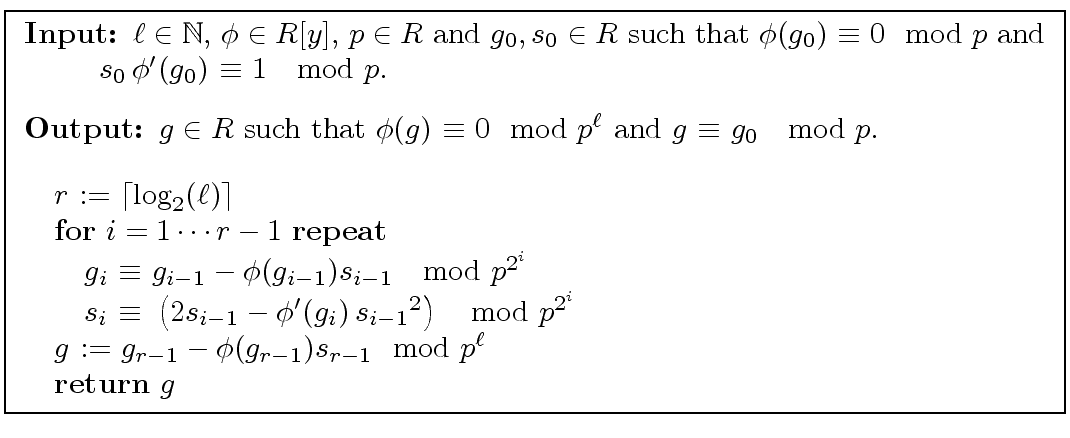Theorem 3   Algorithm 1 is correct.

Proof. Let. Then we have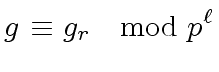(36)

In other wordsis a solution of higher precision. Then proving the theorem turns into proving the invariants for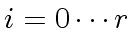1.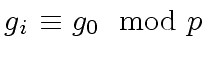,
2.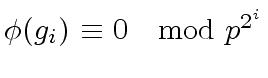,
3. if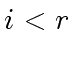then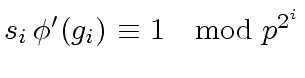.
The case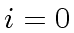is clear and we assume that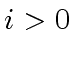holds. Then by induction hypothesis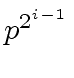divides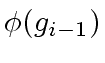and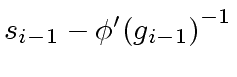. Then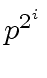divides their product, that is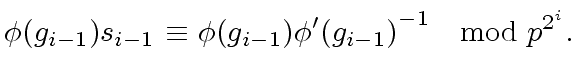(37)

Now we calculate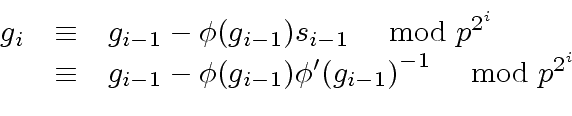(38)

Now applying Proposition 8 with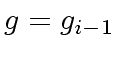,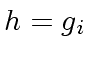and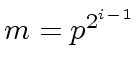we obtain the first two invariants. In addition, this leads to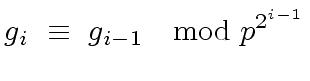(39)

for. This implies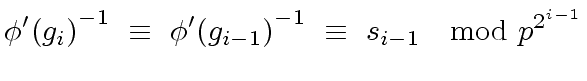(40)

Now,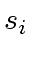is obtained by one Newton step for inversion (see Algorithm). Therefore the third invariant follows.Marc Moreno Maza
2008-01-07